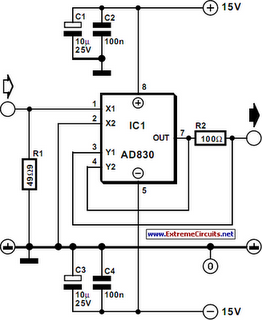# Learning Electronics

Learn to build electronic circuits

# Fast Voltage-Driven Current Source

The current source in the diagram, which react very fast to changes in the input signal, may be used, for instance, in certain measurements. Differential amplifier IC1 ensures that the potential across R2 is equal to the input voltage: Iout =Uin/R2. The bandwidth, B, is given by B=R2 f /RL, where f=80 MHz, and the load impedance RL≥ R2 (both in ohms). The input is terminated into R1 to give the usual 50Ω impedance required by measuring instruments. At the same time, this resistor sets the d.c. operating point. If the link to the driving signal source is short and d.c. coupled, R1 may be omitted. The peak voltage between pins 1 and 2 of the IC is limited to 2.1 V to prevent too large a current at the output. Therefore, the peak output current is 2.1/100=21 mA.

Circuit diagram:Fast Voltage-Driven Current Source Circuit Diagram
Author: H. Bonekamp